Difference between revisions of "Inverse temperature"

It is often convenient to define a dimensionless inverse temperature,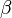$\beta$: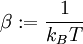$\beta := \frac{1}{k_BT}$

This notation likely comes from its origin as a Lagrangian multiplier, for which Greek letters are customarily written.

Indeed, it shown in Ref. 1 that this is the way it enters. The task is to maximize number of ways $N$ particles may be asigned to $K$ space-momentum cells, such that one has a set of occupation numbers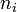$n_i$. Introducing the partition function: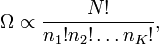$\Omega\propto\frac{N!}{n_1! n_2! \ldots n_K!} ,$

one could maximize its logarithm (a monotonous function):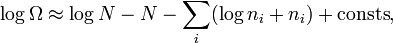$\log \Omega \approx \log N -N - \sum_ i ( \log n_i + n_i) + \mathrm{consts} ,$

where Stirling's approximation for large numbers has been used. The maximization must be performed subject to the constraint: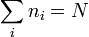$\sum_i n_i=N$

An additional constraint, which applies only to dilute gases, is: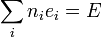$\sum_i n_i e_i=E$,

where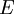$E$ is the total energy and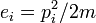$e_i=p_i^2/2m$ is the energy of cell$i$.

The method of Lagrange multipliers entail finding the extremum of the function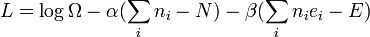$L=\log\Omega - \alpha (\sum_i n_i - N ) - \beta ( \sum_i n_i e_i - E )$,

where the two Lagrange multipliers enforce the two conditions and permit the treatment of the occupations as independent variables. The minimization leads to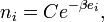$n_i=C e^{-\beta e_i},$

and an application to the case of an ideal gas reveals the connection with the temperature,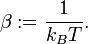$\beta := \frac{1}{k_BT} .$